## Visualizing the similarity of two networks

December 9, 2016

Julia Len (jlen at ucsd.edu)

### Introduction

When working with networks, it is often useful to consider how similar two networks are.  There are a number of ways of quantifying network similarity however.  One could simply consider the number of nodes two networks have in common.  However, this would miss any structural similarity, or lack thereof, between the edges.  For example, it is possible for two networks to have completely identical node sets, but have completely disjoint edge sets.  Note however that in order for two networks to share edges, they must share nodes as well (since edges are defined by the nodes they connect).

In this post, we will introduce a network overlap visualization function (draw_graph_union) in the visJS2jupyter package, and explore a few possible scenarios.

### Installation

To install visJS2jupyter, run

`    pip install visJS2jupyter`

in your terminal. To import the visualizations module, use the statement

`    import visJS2jupyter.visualizations as visualizations`

in your jupyter notebook. The source code is also available on github here.

### Simple example with default parameters

We will now go through a simple example using two 10-node networks whose intersection is exactly 5 nodes. We create two small, random networks using the networkx function ‘connected_watts_strogatz_graph’. Each network will have 10 nodes, with each node initially connected to its 5 nearest neighbors. These connections are then randomly rewired with probability 0.1.

`    G1 = nx.connected_watts_strogatz_graph(10,5,.1)`

`    G2 = nx.connected_watts_strogatz_graph(10,5,.1)`

This produces two networks who both have nodes labelled from 0 to 9. Their intersection is then all the nodes for each graph. This is an unexciting case, so let’s relabel some nodes, so that they share only 5 nodes in common. We can do this by relabelling the nodes 0 to 9 of the second graph, G2, to 5 to 14 using the networkx function ‘relabel_nodes’. The code for this is shown below:

`    old_nodes = range(5)`

`    new_nodes = range(10,15)`

`    new_node_labels = dict(zip(old_nodes,new_nodes))`

`    G2 = nx.relabel_nodes(G2,new_node_labels)`

Now nodes 0 to 4 belong to only G1, nodes 5 to 9 belong to G1 and G2, and nodes 10 to 14 belong to only G2. Let’s see what this looks like by using draw_graph_union:

`    visualizations.draw_graph_union(G1,G2)`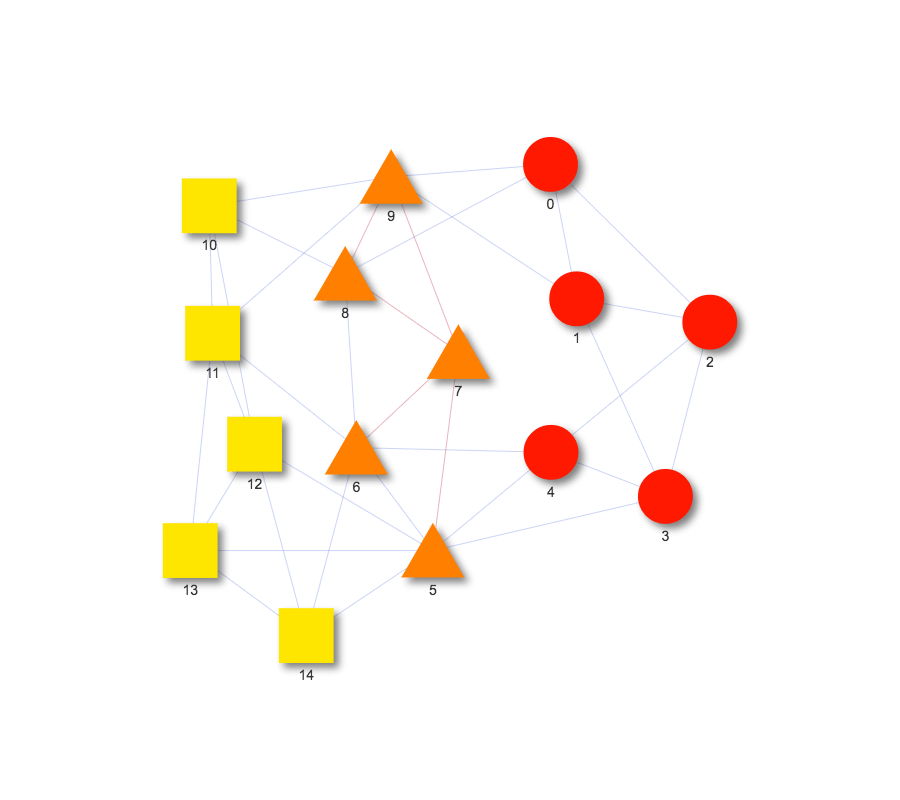And that’s it! We get an interactive graph fairly quickly and easily. Notice that the nodes are color-coded and shaped based on which network they belong to. For instance, nodes in the intersection of G1 and G2 are orange and triangular shapes, while nodes which only belong to G1 are red circles, and nodes which only belong to G2 are yellow squares. Also notice that edges found in both G1 and G2 are colored red while all other edges are colored blue.

You can take a look at the sample notebook here.  Notice that hovering over a node pops up a tooltip with information about the node’s name and graph membership.

From the previous example, we saw that draw_graph_union not only depicts the intersection of nodes, but it also visualizes the intersection of edges as well. Let’s take a look at how this works with two networks having identical nodes but but only a few overlapping edges.

### Identical nodes, some overlapping edges

We’ll again be using the connected_watts_strogatz_graph to create our two networks, but this time both networks will contain 50 nodes:

`    G1 = nx.connected_watts_strogatz_graph(50,5,.1)`

`    G2 = nx.connected_watts_strogatz_graph(50,5,.1)`

This produces two networks with identical nodes and randomly intersecting edges. We want the sets of edges to only intersect over 5 nodes. Python’s built-in set object can help with this. We can get the edges for G1 and G2, convert the lists of edges to sets of edges, and then find their intersection using &. We can then subtract out the intersecting edges from each set of edges.

`    edges_1 = set(G1.edges())`

`    edges_2 = set(G2.edges())`

`    intersecting_edges = edges_1 & edges_2`

`    edges_1_disjoint = edges_1 - intersecting_edges`

`    edges_2_disjoint = edges_2 - interesecting_edges`

This produces two disjoint sets of edges. We now want to add back in 5 edges from the intersection into each disjoint set.

`    for i in range(0,5):`

`        new_edge = intersecting_edges.pop()`

`        edges_1_disjoint.add(new_edge)`

`        edges_2_disjoint.add(new_edge)`

We can then remove the current edges from G1 and add back in the desired edges. We do the same for G2.

`    G1.remove_edges_from(edges_1)`

`    G1.add_edges_from(list(edges_1_disjoint))`

Let’s now draw the two graphs using draw_graph_union, but this time let’s customize the graph a bit. The function sets the default color of the nodes to matplotlib’s colormap autumn and the default color of the edges to matplotlib’s colormap coolwarm. However, matplotlib has many wonderful colormaps available that we can choose from (click here for more details). To set the colormap of the nodes and edges, use the arguments node_cmap and edge_cmap. If you decide to change the colormap, make sure to import the matplotlib package:

`    import matplotlib as mpl`

We can add other customizations as well, such as setting edge width and edge shadows. The function allows for any argument available in visJS_module in the visJS2jupyter package. This allows many potential customizing features for the function. Now, let’s see what this looks like overall:

`    visualizations.draw_graph_union(G1,G2,`

`        node_cmap=mpl.cm.cool,`

`        edge_cmap=mpl.cm.winter_r,`

`        edge_width=5,`

`        edge_shadow_enabled=True,`

`        edge_shadow_size=2)`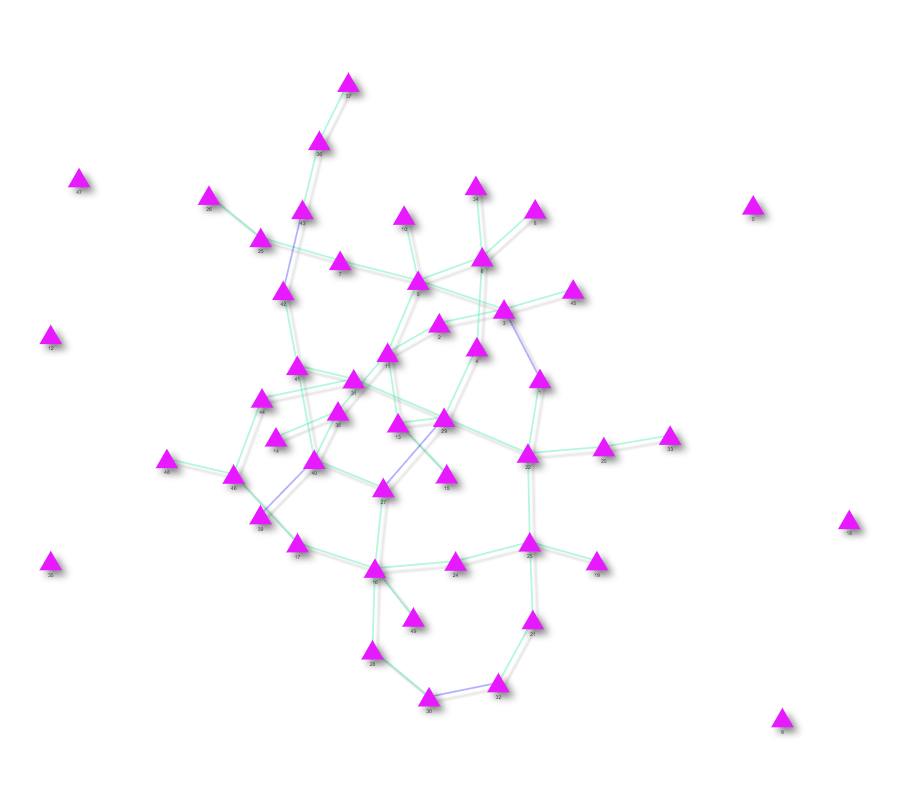As you can see in the graph above, there is now only one set of nodes, all of which are triangle shaped because all the nodes overlap. The edges are mostly colored in green except for 5 edges in blue: the edges in the intersection. Notice that the edges and nodes are colored differently from before and the edges now have added shadows. You can take a look at the interactive notebook with this example here.

### One network contains the other network

So far, we’ve seen graphs where there is a small intersection of the nodes and the node sets are equal. What happens if the set of nodes for one graph is a subset of the nodes for the other graph? We’ll take a look at this case now.

We again create two networks using connected_watts_strogatz. Graph 1 will have 50 nodes and graph 2 will have 20 so that all of the second graph’s nodes intersect with graph 1. We will then call draw_graph_union on these two graphs. This time, we use some more features of the function. We can set the name of the nodes for graph 1 and graph 2. Notice that previously when we hovered over a node, the tooltip showed something like “graph 1 + graph 2”. Using the arguments node_name_1 and node_name_2, we can customize what is shown in the tooltip. Pretty cool!

If you’ve played around with some of the example notebooks, you’ve probably noticed that the nodes move around when dragged as if they have a gravitational field. This is the physics_enabled feature. It is set by default for graphs of less than 100 nodes, while it is turned off for any larger graphs. One nice feature is that you can override this by setting the physics_enabled argument to true or false. Let’s turn off this setting for this example.

`    visualizations.draw_graph_union(G1,G2,edge_width=5`

`        node_name_1=”superset”,`

`        node_name_2=”subset”,`

`        physics_enabled=False)`In just a couple of lines of code, we have produced an interactive network! Notice that when we hover over a node, it has the name that we set, just like we wanted. You can also see that dragging a node around makes it stay stuck in place, so the physics_enabled setting has been turned off. The example notebook can be found here.

Overall, draw_graph_union provides a quick and easy way to create customizable and interactive visualizations for network similarities, enabling visual assessment of what two networks share and what they don’t.

## Communities and cliques

November 4, 2016

Brin Rosenthal (sbrosenthal at ucsd.edu)

You probably won’t get far learning about networks and graph theory before coming across communities and cliques in graphs. At first glance, these two concepts are quite similar- they both describe highly connected sets of nodes, after all. There are however situations which are best suited to one or the other. In this post we will explore some similarities and differences between communities and cliques, and a specific problem I came upon which I thought would be easily solved by a community-finding algorithm, but soon realized that cliques were the much better option!

A network community is a set of nodes which have dense connections within the group, and sparse connections outside the group. A large number of community finding algorithms have been developed, which vary in complexity, and have different strengths and weaknesses depending on context, of which we list a small sampling: Fortunato, S, Malliaros, F, Newman, M., Airoldi, E. et al, Karrer, B., Newman, M., Peixoto, T., Johnson, S.. The community-finding algorithms generally optimize some parameter (usually related to the number of within-group and between-group edges). They are also generally stochastic, meaning that you may get slightly different answers on different runs. It can be useful to explore the community structure of many real-world networks, to establish substructure in the graph. Unlike cliques, there are no exact communities in a graph, rather you will get different answers depending on what algorithm you use, and what you are optimizing for.

A clique is in some sense a stronger version of a community. A set of nodes forms a clique (equivalently, a complete subgraph) if all possible connections between nodes exist. A two-node clique is simply two connected nodes. A three node clique is also known as a triangle. Graphs also contain maximal cliques, which are complete subgraphs such that no other node can be added while maintaining completeness. In Figure 1 below, there are only two maximal cliques of size greater than two. There is a maximal clique of size 5 (orange nodes), and a maximal clique of size 3 (green nodes).Figure 1: Nodes color-coded by clique-membership.

One of the most commonly used maximal clique-finding algorithms may be found here, and recursively searches through the graph finding all maximal cliques. Depending on structure, graphs may contain a large number of cliques, resulting in a high memory cost for the algorithm.

In my research I have mainly focused on applications of community-finding algorithms to biological networks. However, I recently came upon a problem that was solved much better using a clique-finding approach than a community-finding one. Briefly, we had a matrix of similarity between objects (Figure 2), and we wanted to find sets of objects which were all very dissimilar from each other.Figure 2: Randomly generated similarity scores between 100 objects

Application of community-finding algorithms resulted in either groups of highly similar objects grouped together (modularity maximization- Figure 3), or groups of objects which were mostly dissimilar, but contained a couple similar objects in the group. In our application, we couldn’t tolerate any similar objects in our groups, so both of these solutions weren’t satisfactory.Figure 3: Objects clustered by dissimilarity, using modularity maximization algorithm. Groups are only weakly dissimilar, with many similar pairs existing in the same groups.

I then realized that I could reform the problem of finding sets of highly dissimilar objects into a clique-finding framework. I simply created a network from our similarity matrix by connecting nodes whenever they had a similarity level less than a certain tolerance. Once this dissimilarity network was created, it was simply a matter of applying the networkx function find_cliques to the graph. In our network of 100 nodes and 649 edges, there were 362 maximal cliques, with the largest of these maximal cliques containing 4 nodes.

While most community-finding algorithms discretely partition graphs (with some exceptions), nodes can belong to a large number of maximal cliques (see example below- Figure 4). For our problem, this meant we had a large number of maximal cliques to choose from, but many of them contained a lot of the same information.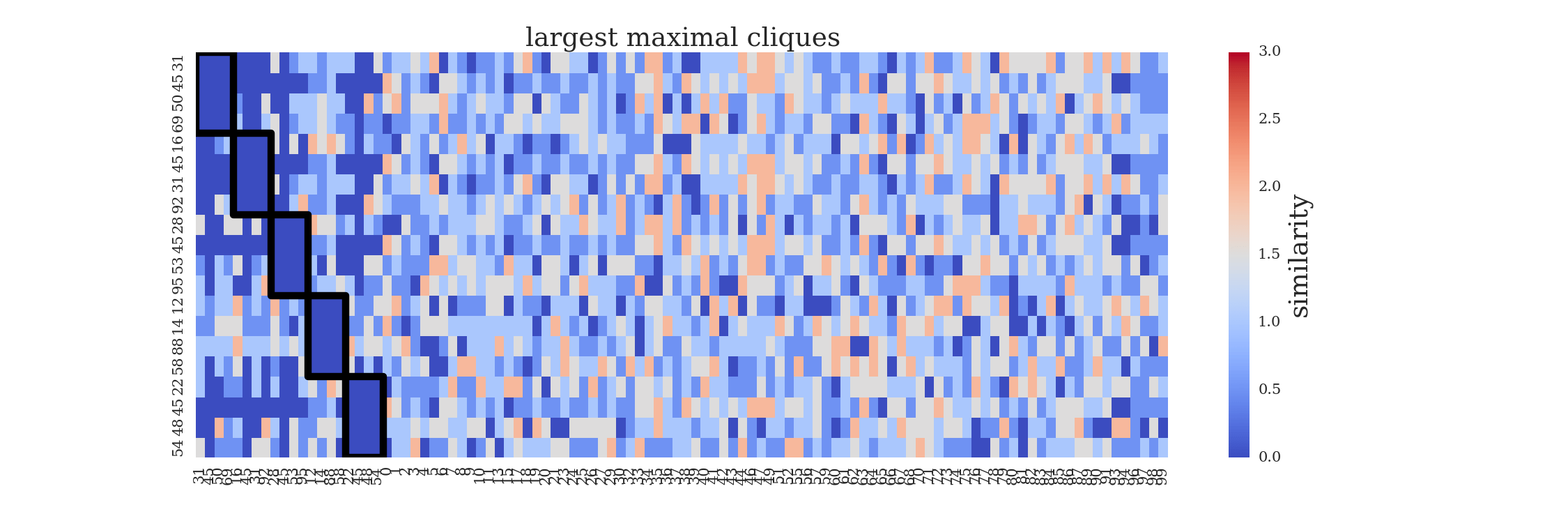Figure 4: Five largest maximal cliques outlined in black.

We can tune the sizes of our dissimilar sets by changing the threshold of similarity we can tolerate. When we increase the similarity threshold from 0.0 to 1.0, we now have 2840 maximal cliques, and the largest maximal clique contains 6 nodes (Figure 5).Figure 5: Cliques formed with sets of nearly perfectly dissimilar objects.

That’s about all for this post, although please note we have barely scratched the surface of communities and cliques in graphs. Please see the links for more in-depth reading!

## Bringing interactivity to network visualization in Jupyter notebooks: visJS2Jupyter

September 30, 2016

Brin Rosenthal (sbrosenthal at ucsd.edu)

### Introduction

Data is everywhere these days, and being able to interact with visual representations of that data in real time can help bring it to life.  You have to look no further than the D3 (data-driven-documents) examples page to see this.  If you haven’t spent time browsing through the D3 examples library, I would highly recommend doing so, but be warned it is easy to spend a few captivating hours here! (A few of my favorites: collision avoidancecollapsible force layout,  NCAA march madness predictionspreferential attachment).

Unfortunately, D3 is pretty nontrivial to learn, which can be a significant barrier to those of us looking for a quick but awesome solution.  There are some good visualization libraries which are based on D3, and simpler to use.  One of our favorites is vis.js.

If you’re anything like me, you love the fast and flexible development and documentation environment that Jupyter notebooks provide.  But I had been frustrated with the limited interactivity that is available for plotting of data.  While matplotlib, seaborn, and networkx provide nice static ways of graphing data and networks, they left me wanting more.  Python widgets are ok, but a bit clunky (see earlier post…) .

A group of us at the CCBB had the idea to write a tool which would bring the interactivity of D3 (through vis.js) into Jupyter notebook cells.  This turned out to be quite simple.  We repurposed some existing html code from another project, to set the styles of nodes and edges in a network.  We modified this code to allow style arguments to be passed in through a function.  Every time this function is called, a new style_file.html is created, containing the properties set by the user.  This style_file.html is then loaded into the Jupyter cell using the python HTML module, and the network is rendered in the cell.  Once we figured these pieces out, we had a fully interactive graph!  Right there in the Jupyter notebook cell!  We can now freely pan, zoom, click and drag nodes, and even embed more information in the node and edge hover-bubbles.  One of the coolest things about this tool is that it is almost infinitely flexible, and we’ve designed it to work with networkx graph formats- are one of the most standard python graph libraries.

In this post, I’ll walk you through two simple examples of how to use visJS2Jupyter.

### Installation

To install, run “pip install visJS2jupyter” in your terminal. To import, use the statement “import visJS2jupyter.visJS_module” in your notebook.  Source code for the package may be found here https://github.com/ucsd-ccbb/visJS_2_jupyter.

### Use example with default parameters

Now that we have the package installed, we’re going to walk through a very simple use example, using only the default parameters.  First, we need a network to draw.  Let’s make a random one using the networkx function ‘connected_watts_strogatz_graph’.  This network has 30 nodes, each of which is initially connected to 5 nearest neighbors.  Each of these connections randomly rewired with probability 0.2.  We will also need the lists of nodes and edges that comprise this graph.

``````
G=nx.connected_watts_strogatz_graph(30,5,.2)
nodes = G.nodes()
edges = G.edges()
``````

Next, we will simply construct dictionaries which contain all of the node-specific and edge-specific traits which will be passed to the visualizer.  (Note that we also need to make a node_map here, which maps the names of the nodes in the graph to integers, because of the way visJS interprets node/edge data).

``````    nodes_dict = [{"id":n} for n in nodes]
node_map = dict(zip(nodes,range(len(nodes)))) # map to indices for source/target in edges
edges_dict = [{"source":node_map[edges[i]], "target":node_map[edges[i]],
"title":'test'} for i in range(len(edges))]

``````

Now all that’s left is calling the visualizer function:

``````
visJS_module.visjs_network(nodes_dict, edges_dict, time_stamp=0)

``````

Done! Now we are free to click, drag, and zoom at will. Note that if you click on a node, that node’s nearest neighbors are highlighted.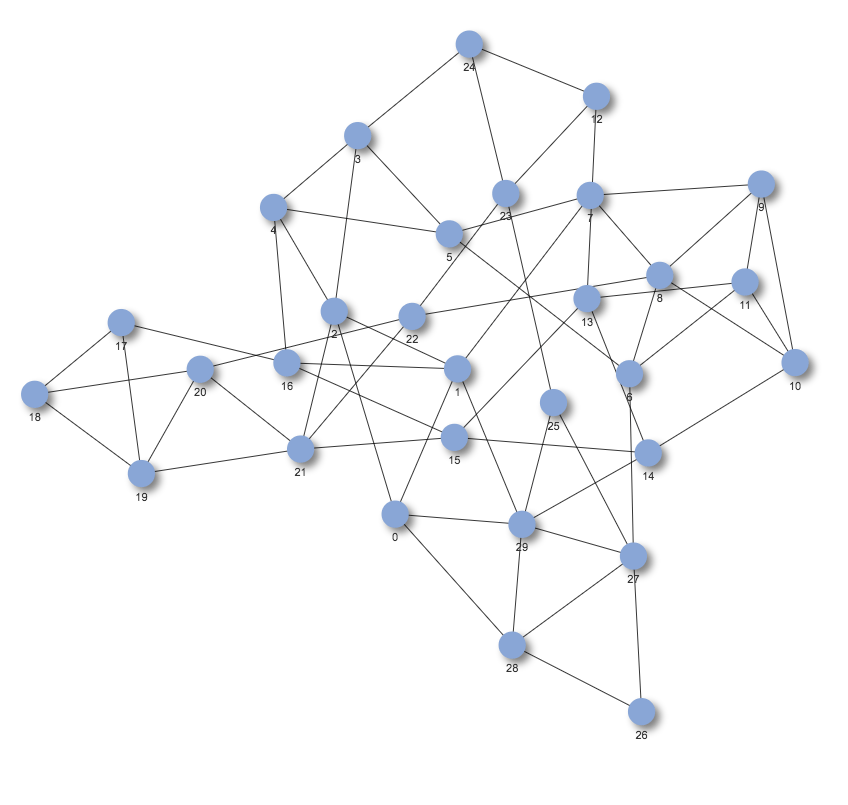Now that we have the basic use example under our belt, let’s move on to something more complicated, because there is so much potential here!

### More complicated use example

In this example, we will start by mapping some features to node and edge properties.  To map node/edge attributes to properties, simply add the property to the graph as a node/edge-attribute (using nx.set_node_attribute and nx.set_edge_attribute), then use the return_node_to_color function to select which property you would like to map to the node colors.  You can map anything you want to node color, as long as you represent it numerically.  You can also choose which matplotlib colormap  you’d like to use for the mapping.  For example, let’s calculate the node-level clustering coefficient and betweenness centrality and degree for our random network we made above, and add them as attributes.

``````
# add a node attributes to color-code by
cc = nx.clustering(G)
degree = G.degree()
bc = nx.betweenness_centrality(G)
nx.set_node_attributes(G,'clustering_coefficient',cc)
nx.set_node_attributes(G,'degree',degree)
nx.set_node_attributes(G,'betweenness_centrality',bc)
``````

Now that we’ve added each of these properties as node attributes, let’s map the node colors to betweenness centrality, and use the matplotlib colormap spring_r for our color scheme. We can also set the node transparency, using alpha, (1 = fully opaque, 0 = fully transparent), and we can choose which section of the colormap we’d like to use. Here we’re setting the lowest value of betweenness centrality to 10% of spring_r, and the highest value to 90%. This is useful if you like most of a colormap, but only want to use the part you like (if it starts too light or too dark for example). You can also transform your color scale, using the ‘color_vals_transform’ argument. Valid options are ‘log’, ‘sqrt’, and ‘ceil’.

``````
node_to_color =   visJS_module.return_node_to_color(G,field_to_map='betweenness_centrality',cmap=mpl.cm.spring_r,
alpha = 1, color_max_frac = .9,color_min_frac = .1)

``````

Now that we have our color mapping, we can fill out nodes_dict, node_map, and edges_dict, as we did in the simple example. This time, however, we will set more node and edge level properties, including:

• the positions of each node (x and y) using the output from nx.spring_layout
• The color of each node using our color mapping node_to_color
• The degree of each node (if degree is passed in, it is used to map node size by default)
• We’ll pass in dummy values for the node title field (this is what will show up in the hover).
• The color of each edge (for now we set every edge to be the same color- gray, but you can easily individualize the edge colors too, using visJS_module.return_edge_to_color(…)).

This is the current list of properties you can modify at the node level

• ‘node_shape’
• ‘color’
• ‘border_width’
• ‘title’ (e.g. the hover information)
• The default node size is mapped to the node degree, but you can override that default by setting ‘node_size_field’ in the visjs_network function.  For example, simply add a ‘node_size’ key:value entry to the nodes_dict, and call visjs_network with node_size_field = ‘node_size’.
• ‘degree’: the degree of each node- used for default size mapping
• All of the above are optional additions to nodes_dict.  Default values will be filled in if they are missing.

``````
pos = nx.spring_layout(G)
nodes_dict = [{"id":n,"color":node_to_color[n],
"degree":nx.degree(G,n),
"x":pos[n]*1000,
"y":pos[n]*1000} for n in nodes
]
node_map = dict(zip(nodes,range(len(nodes))))  # map to indices for source/target in edges
edges_dict = [{"source":node_map[edges[i]], "target":node_map[edges[i]],
"color":"gray","title":'test'} for i in range(len(edges))]

``````

We’ll also pass in some more graph-level properties (properties that aren’t node and edge specific). These include:

• node_size_multiplier: multiply each node’s size by this (useful if you have very few or very many nodes)
• node_color_highlight_border
• node_color_highlight_background
• node_color_hover_border
• node_color_hover_background
• node_font_size
• edge_arrow_to: Should we draw arrows at the target end?
• edge_color_highlight
• edge_color_hover
• edge_width: how wide should the edges be?
• physics_enabled, min_velocity, max_velocity: controls the physics of the nodes
• Time_stamp: This appends the value to the end of the style-file, thus creating a new one instead of writing over the old one.  You need a unique style-file for every network you render within the same Jupyter notebook.

We have mapped most (still working on getting the complete list) of the modifiable fields from visJS network into our package.  You can find documentation on the full list here .

``````
visJS_module.visjs_network(nodes_dict,edges_dict,time_stamp=1,
node_size_multiplier=5,
node_size_transform = '',
node_color_highlight_border='red',
node_color_highlight_background='#D3918B',
node_color_hover_border='blue',
node_font_size=25,
edge_arrow_to=True,
edge_color_highlight='#8A324E',
edge_width=3,
physics_enabled=True,
min_velocity=1,
max_velocity=15)
``````

Ok there we go! Now we have drawn a much more interesting network.  Click on the image below to be redirected to the interactive version, hosted on bl.ocks.org.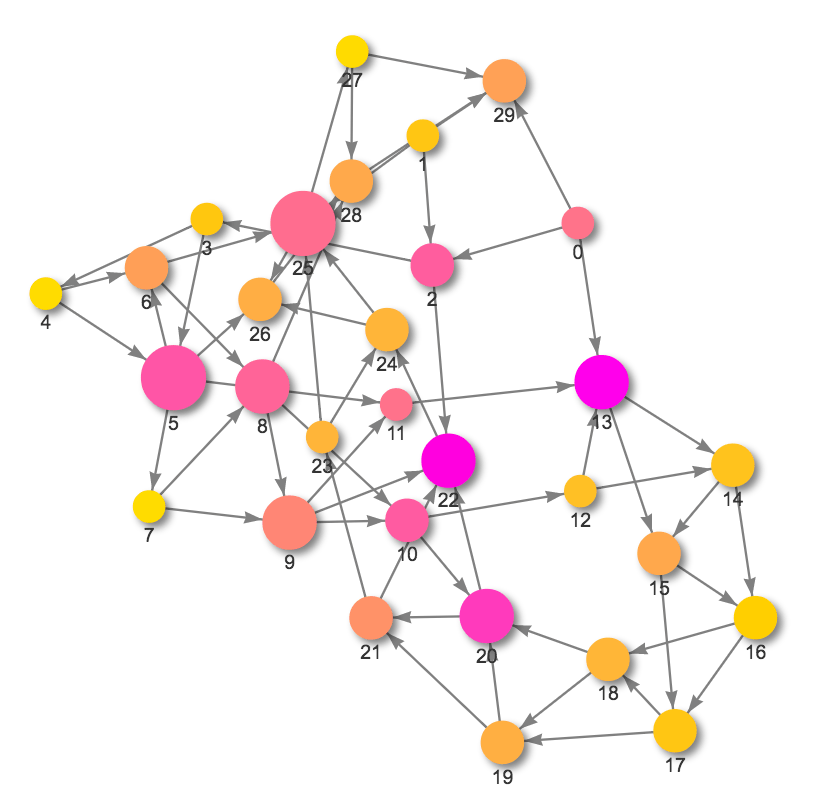For an even more complicated use case, see this notebook I wrote (http://bl.ocks.org/brinrosenthal/raw/fd7d7277ce74c2b762d3a4d66326215c/).  In this example, we display the bipartite network composed of diseases in The Cancer Genome Atlas (http://cancergenome.nih.gov/), and the top 25 most common mutations in each disease. We also overlay information about drugs which target those mutations. Genes which have a drug targeting them are displayed with a bold black outline. The user may hover over each gene to get a list of associated drugs.

## Visualize and analyze differential expression data in a network

December 16, 2015

In analysis of differential expression data, it is often useful to analyze properties of the local neighborhood of specific genes. I developed a simple interactive tool for this purpose, which takes as input diferential expression data, and gene interaction data (from http://www.genemania.org/). The network is then plotted in an interactive widget, where the node properties, edge properties, and layout can be mapped to different network properties. The interaction type (of the 6 options from genemania) can also be selected.

This notebook will also serve as an example for how to create, modify, visualize and analyze weighted and unweighted gene interaction networks using the highly useful and flexible python package NetworkX (https://networkx.github.io/)

This tool is most useful if you have a reasonably small list of genes (~100) with differential expression data, and want to explore properties of their interconnections and their local neighborhoods.

The interactive ipython notebook version of this post may be accessed here (https://github.com/brinrosenthal/DE_network_visualizer), where you can use the network visualizer with our example data, or insert your own data.

### Import a real network (from this experiment http://www.ncbi.nlm.nih.gov/sites/GDSbrowser?acc=GDS4419)¶

This experiment contains fold change information for genes in an experiment studying ‘alveolar macrophage response to bacterial endotoxin lipopolysaccharide exposure in vivo’. We selected a list of genes from the experiment which had high differential expression, and were enriched for ‘immune response’ and ‘response to external biotic stimulus’ in the gene ontology. This experiment and gene list were selected purely as examples for how to use this tool for an initial exploration of differential expression data.

### Description of options:¶

• focal_node_name: Select gene to focus on (a star will be drawn on this node)
• edge_threshold: Change the number of edges included in the network by moving the edge_threshold slider. Higher values of edge_threshold means fewer edges will be included in the graph (and may improve interpretability). The threshold is applied to the ‘Weight’ column of DE_network, so the less strongly weighted edges are not included as the threshold increases
• network_algo: Select the network algorithm to apply to the graph. Choices are:
• ‘spl’ (shortest path length): Plot the network in a circular tree layout, with the focal gene at the center, with nodes color-coded by log fold-change.
• ‘clustering coefficient’: Plot the network in a circular tree layout, with nodes color-coded by the local clustering coefficient (see https://en.wikipedia.org/wiki/Clustering_coefficient).
• ‘pagerank’: Plot the network in a spring layout, with nodes color-coded by page rank score (see https://en.wikipedia.org/wiki/PageRank for algorithm description)
• ‘community’: Group the nodes in the network into communities, using the Louvain modularity maximization algorithm, which finds groups of nodes optimizing for modularity (a metric which measures the number of edges within communities compared to number of edges between communities, see https://en.wikipedia.org/wiki/Modularity_(networks) for more information). The nodes are then color-coded by these communities, and the total modularity of the partition is printed above the graph (where the maximal value for modularity is 1 which indicates a perfectly modular network so that there are no edges connecting communities). Below the network the average fold-change in each community is shown with box-plots, where the focal node’s community is indicated by a white star, and the colors of the boxes correspond to the colors of the communities above.
• map_degree: Choose whether to map the node degree to node size
• plot_border_col: Choose whether to plot the log fold-change as the node border color
• draw_shortest_paths: If checked, draw the shortest paths between the focal node and all other nodes in blue transparent line. More opaque lines indicate that section of path was traveled more often.
• coexpression, colocalization, other, physical_interactions, predicted_interactions, shared_protein_domain: Select whether to include interactions of these types (types come from GeneMania- http://pages.genemania.org/data/)

## Some examples¶

First let’s look at the graph when ‘spl’ (shortest path length) is selected as the network algo. ADA is the focal node in this case, and it has 4 nearest neighbors (MX1, CD44, FITM1, and CD80). Note that CD44 connects the focal node ADA to many other nodes in the network, as it is an opaque blue line. Also note that there is only one gene with anegative fold change in this gene set (CCL13). The white nodes are genes included by genemania- they are the 20 genes nearest to the input genelist.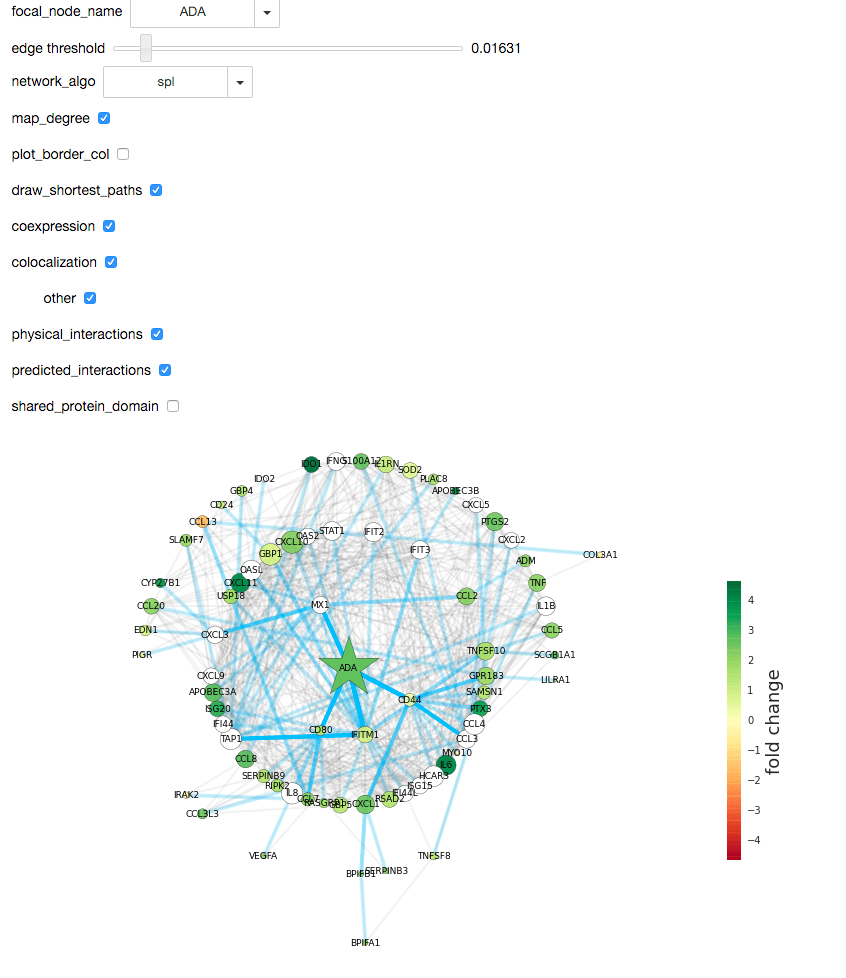### Community detection¶

When the network_algo button is switched to ‘community’, the louvain modularity maximization algorithm runs on the network, and partitions the nodes into communities which maximize the modularity. In this case (with CXCL10 as the focal node), the nodes are partitioned into 5 groups, with the three largest groups indicated by red, green, and teal circles. While you can see some support for this grouping by eye, the overall graph modularity is 0.33, which is a relatively low value. This means that although groups were found in the graph, the graph itself is not very modular. As a rule of thumb, very modular graphs have modularities of about 0.5 or 0.6.Below the graph, there is a panel showing the average fold change for the nodes in this community. Since most of the nodes in the input gene list have positive fold changes here, all communities also have positive average fold changes. Were the input gene list to have fewer large fold changes, this would enable you to see if a particular grouping of nodes had significantly higher (or lower) levels of differential expression than alternative groupings.